﻿ Table of Cross Section Properties | MechaniCalc

The table below gives properties of common cross sections. More extensive tables can be found in the listed references.

The properties calculated in the table include area, centroidal moment of inertia, section modulus, and radius of gyration. For information on cross section properties, see our on cross section properties reference.

Shape Representation Properties
Rectangle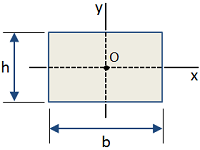Area [in2]:

 A = bh

Moment of Inertia [in4]: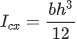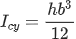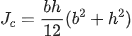Section Modulus [in3]: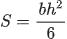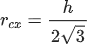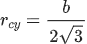Circle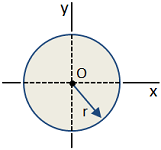Area [in2]: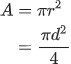Moment of Inertia [in4]: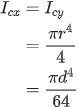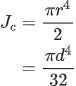Section Modulus [in3]: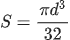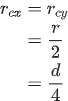Circular Tube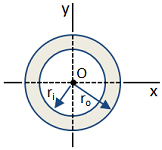Area [in2]: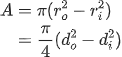Moment of Inertia [in4]: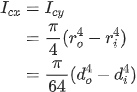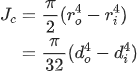Section Modulus [in3]: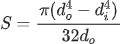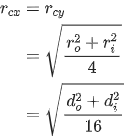I-Beam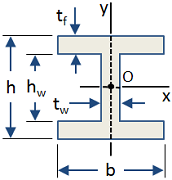Area [in2]: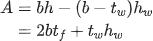Moment of Inertia [in4]: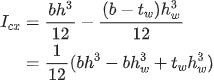Section Modulus [in3]: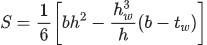#### Need Structural Calculators?

We have a number of structural calculators to choose from. Here are just a few: